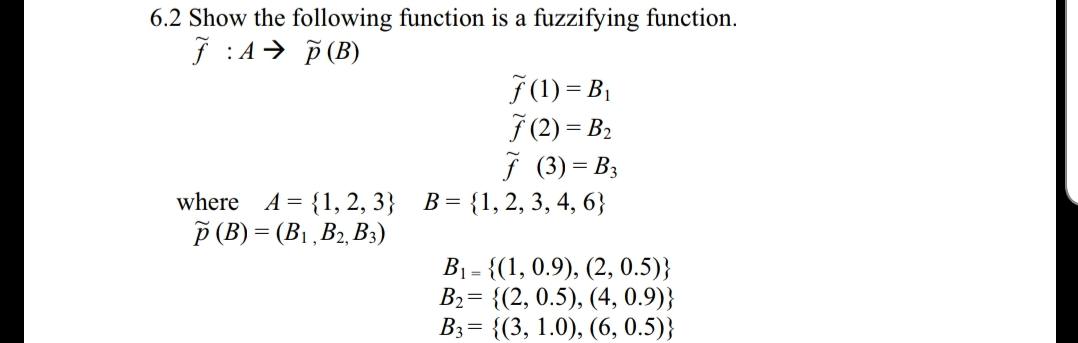# 6.2 Show the following function is a fuzzifying function.J A (B)f (1) = B70) = B2f (3) BA-1,2,3 B-11,2, 3, 4, 6where1 , D2, D3B1-1, 0.9), (2, 0.5))B2 2, 0.5), (4, 0.9)]B,-{(3, 1.0), (6,0.5)}

Question
42 views

the book is "First Course on Fuzzy Theory and Applications"help_outlineImage Transcriptionclose6.2 Show the following function is a fuzzifying function. J A (B) f (1) = B 70) = B2 f (3) B A-1,2,3 B-11,2, 3, 4, 6 where 1 , D2, D3 B1-1, 0.9), (2, 0.5)) B2 2, 0.5), (4, 0.9)] B,-{(3, 1.0), (6,0.5)} fullscreen
check_circle

Step 1

To show that the given assignment f(tilda) is a fuzzifying function

Step 2

Definition: A fuzzifying function from A to B is just a function from A to a fuzzfied P(B), (the power set of B)

Step 3

Recall the definition of an (ordin...

### Want to see the full answer?

See Solution

#### Want to see this answer and more?

Solutions are written by subject experts who are available 24/7. Questions are typically answered within 1 hour.*

See Solution
*Response times may vary by subject and question.
Tagged in

### Math